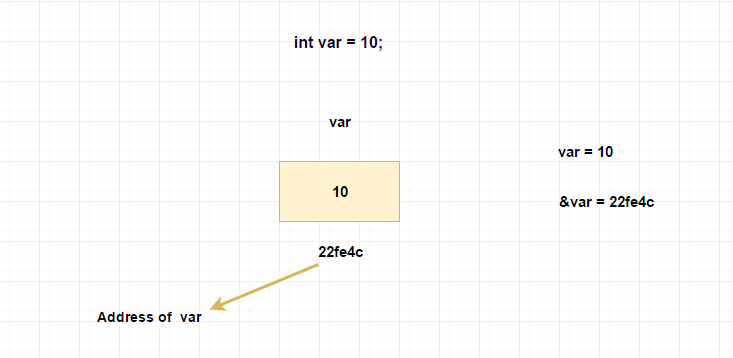# scanf function

When the c program needs to get input from the user, we should use scanf function.

To get input from the user, we should instruct the scanf function the following details,

Specify the format i.e. which data type we are going to get from the user.

Give the variable address where we want to store the value.

## Syntax

```scanf("<format specifier>" , <address of variable>);
```

To know about format specifiers kindly follow the below link,

Format specifiers in C

## How to get the address of a variable ?

Using ampersand (&) operator, we can get the address of a variable. In C, we will refer & as address of operator.

#### Pictorial ExplanationIn scanf, we must give the address of a variable not variable name.

## Printing address of a variable

Example

```#include<stdio.h>

int main()
{
int var = 10;

printf("value of var = %d \n",var);
printf("Address of var = %x \n",&var);

return 0;
}
```

%x is used to print the address in hexadecimal format.

## Sample Program

This program will get values from the user and print it.

Example

```#include<stdio.h>

int main()
{
int   age;
float weight;

//getting input from the user and storing it into memory addresses
scanf("%d%f",&age,&weight);

printf("age = %d \nweight = %f \n",age,weight);

return 0;
}
```

## Correct the below program

The below program has some syntax errors.

Correct the program by yourself and see the output.

Example

```#include<stdio.h>

int main()
{
int i;

printf("Enter any number\n");

//correct the below statement
scanf("%d",i);

printf("i = %d\n",i);

return 0;
}
```

## Useful Resources

https://linux.die.net/man/3/scanf
https://en.wikipedia.org/wiki/Scanf_format_string